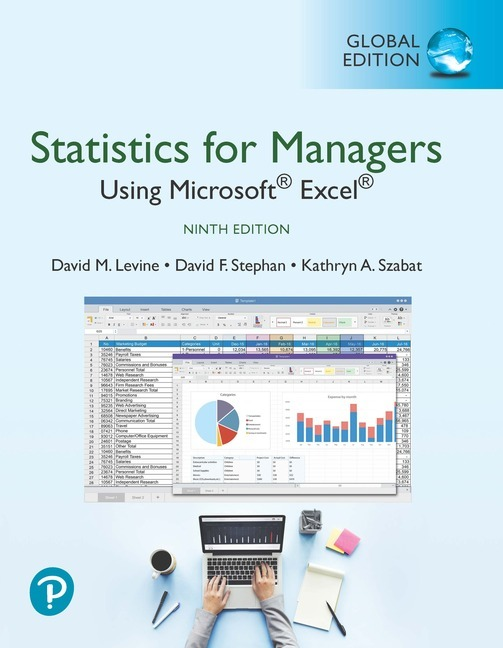• Create your account, and log-in before ordering. Enable to track your order.
• Public Holiday Announcement: We are away on 21st Sat. We'll be back to serve you on 25th Wed.
•# Statistics for Managers Using Microsoft Excel, 9th Edition

RM 100.00
- +

• David M. Levine
• David F. Stephan
• Kathryn A. Szabat
• 9781292338248

courses in Introduction to Business Statistics.

The gold standard in learning Microsoft Excel for business statistics

Statistics for Managers Using Microsoft® Excel®9th Edition, Global Edition helps students develop the knowledge of Excel needed in future careers. The authors present statistics in the context of specific business fields, and now include a full chapter on business analytics. Guided by principles set forth by ASA’s Guidelines for Assessment and Instruction (GAISE) reports and the authors’ diverse teaching experiences, the text continues to innovate and improve the way this course is taught to students. Current data throughout gives students valuable practice analyzing the types of data they will see in their professions, and the authors’ friendly writing style includes tips and learning aids throughout.

Preface

First Things First

FTF.2 Business Analytics: The Changing Face of Statistics
FTF.3 Starting Point for Learning Statistics
FTF.4 Starting Point for Using Software
FTF.5 Starting Point for Using Microsoft Excel

1. Defining and Collecting Data

1.1 Defining Variables
1.2 Collecting Data
1.3 Types of Sampling Methods
1.4 Data Cleaning
1.6 Types of Survey Errors

2. Organizing and Visualizing Variables

2.1 Organizing Categorical Variables
2.2 Organizing Numerical Variables
2.3 Visualizing Categorical Variables
2.4 Visualizing Numerical Variables
2.5 Visualizing Two Numerical Variables
2.6 Organizing a Mix of Variables
2.7 Visualizing a Mix of Variables
2.8 Filtering and Querying Data 73
2.9 Pitfalls in Organizing and Visualizing Variables

3. Numerical Descriptive Measures

3.1 Measures of Central Tendency
3.2 Measures of Variation and Shape
3.3 Exploring Numerical Variables
3.4 Numerical Descriptive Measures for a Population
3.5 The Covariance and the Coefficient of Correlation
3.6 Descriptive Statistics: Pitfalls and Ethical Issues

4. Basic Probability

4.1 Basic Probability Concepts
4.2 Conditional Probability
4.3 Ethical Issues and Probability
4.4 Bayes' Theorem
4.5 Counting Rules

5. Discrete Probability Distributions

5.1 The Probability Distribution for a Discrete Variable
5.2 Binomial Distribution
5.3 Poisson Distribution
5.4 Covariance of a Probability Distribution and Its Application in Finance
5.5 Hypergeometric Distribution

6. The Normal Distribution and Other Continuous Distributions

6.1 Continuous Probability Distributions
6.2 The Normal Distribution
6.3 Evaluating Normality
6.4 The Uniform Distribution
6.5 The Exponential Distribution
6.6 The Normal Approximation to the Binomial Distribution

7. Sampling Distributions

7.1 Sampling Distributions
7.2 Sampling Distribution of the Mean
7.3 Sampling Distribution of the Proportion
7.4 Sampling from Finite Populations

8. Confidence Interval Estimation

8.1 Confidence Interval Estimate for the Mean (σ Known)
8.2 Confidence Interval Estimate for the Mean (σ Unknown)
8.3 Confidence Interval Estimate for the Proportion
8.4 Determining Sample Size
8.5 Confidence Interval Estimation and Ethical Issues
8.6 Application of Confidence Interval Estimation in Auditing
8.7 Estimation and Sample Size Determination for Finite Populations
8.8 Bootstrapping

9. Fundamentals of Hypothesis Testing: One-Sample Tests

9.1 Fundamentals of Hypothesis Testing
9.2 t Test of Hypothesis for the Mean (σ Unknown)
9.3 One-Tail Tests
9.4 Z Test of Hypothesis for the Proportion
9.5 Potential Hypothesis-Testing Pitfalls and Ethical Issues
9.6 Power of the Test

10. Two-Sample Tests

10.1 Comparing the Means of Two Independent Populations
10.2 Comparing the Means of Two Related Populations
10.3 Comparing the Proportions of Two Independent Populations
10.4 F Test for the Ratio of Two Variances
10.5 Effect Size

11. Analysis of Variance

11.1 One-Way ANOVA
11.2 Two-Way ANOVA
11.3 The Randomized Block Design
11.4 Fixed Effects, Random Effects, and Mixed Effects Models

12. Chi-Square and Nonparametric Tests

12.1 Chi-Square Test for the Difference Between Two Proportions
12.2 Chi-Square Test for Differences Among More Than Two Proportions
12.3 Chi-Square Test of Independence
12.4 Wilcoxon Rank Sum Test for Two Independent Populations
12.5 Kruskal-Wallis Rank Test for the One-Way ANOVA
12.6 McNemar Test for the Difference Between Two Proportions (Related Samples)
12.7 Chi-Square Test for the Variance or Standard Deviation
12.8 Wilcoxon Signed Ranks Test for Two Related Populations

13. Simple Linear Regression

13.1 Simple Linear Regression Models
13.2 Determining the Simple Linear Regression Equation
13.3 Measures of Variation
13.4 Assumptions of Regression
13.5 Residual Analysis
13.6 Measuring Autocorrelation: The Durbin-Watson Statistic
13.7 Inferences About the Slope and Correlation Coefficient
13.8 Estimation of Mean Values and Prediction of Individual Values
13.9 Potential Pitfalls in Regression

14. Introduction to Multiple Regression

14.1 Developing a Multiple Regression Model
14.2 Evaluating Multiple Regression Models
14.3 Multiple Regression Residual Analysis
14.4 Inferences About the Population Regression Coefficients
14.5 Testing Portions of the Multiple Regression Model
14.6 Using Dummy Variables and Interaction Terms
14.7 Logistic Regression
14.8 Cross-Validation

15. Multiple Regression Model Building

15.2 Using Transformations in Regression Models
15.3 Collinearity
15.4 Model Building
15.5 Pitfalls in Multiple Regression and Ethical Issues

16. Time-Series Forecasting

16.1 Time-Series Component Factors
16.2 Smoothing an Annual Time Series
16.3 Least-Squares Trend Fitting and Forecasting
16.4 Autoregressive Modeling for Trend Fitting and Forecasting
16.5 Choosing an Appropriate Forecasting Model
16.6 Time-Series Forecasting of Seasonal Data
16.7 Index Numbers

17.2 Descriptive Analytics
17.3 Decision Trees
17.4 Clustering
17.5 Association Analysis
17.6 Text Analytics
17.7 Prescriptive Analytics

18. Getting Ready to Analyze Data in the Future

18.1 Analyzing Numerical Variables
18.2 Analyzing Categorical Variables

19. Statistical Applications in Quality Management (online)

19.1 The Theory of Control Charts
19.2 Control Chart for the Proportion: The p Chart
19.3 The Red Bead Experiment: Understanding Process Variability
19.4 Control Chart for an Area of Opportunity: The c Chart
19.5 Control Charts for the Range and the Mean
19.6 Process Capability
19.7 Total Quality Management
19.8 Six Sigma

20. Decision Making

20.1 Payoff Tables and Decision Trees
20.2 Criteria for Decision Making
20.3 Decision Making with Sample Information
20.4 Utility
Appendices